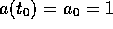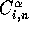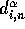Next: The AVP Code Up: Orbits of Galaxies Previous: Orbits of Galaxies

# Implementation of the AVP

As pointed out in Section 4.1, the implementation of the AVP usually starts with Hamilton's principle instead of solving the system of non-linear constraint equations in (3.35). This means that the partial integration in (3.25) is not considered, which in turn means that the boundary values are only being taken account of in the trial functions, (3.22) or (3.23). The basic idea of the AVP is then to find the values of the coefficients in the parameterization of the orbits in equation (3.21) that optimizes the action in equation (4.1). This results in the knowledge of the individual position of each mass tracer in the system at any given point in time, ranging from a=0 until today. If these solutions have any foundation in reality, which, as we shall see later, there are quite a few indications of, this principle will have a wide range of applications. More on this in Section 6.1.

Before getting to the details of the AVP code, let us first sum up some of the more general observations from Chapter 4. For n-dimensional quadratic functions (4.6), the minimum will be located from any starting point in at most n iterations by using any optimizing methods (with the exception of Steepest Descent, which in worst case may use an infinite number of iterations), assuming that there are no numerical discrepancies. With the n-dimensional non-quadratic function studied in this thesis, i.e. the action from equation (4.1), the solutions will at best be reached in n iterations. In addition, the action is non-linear, which may result in a multiple number of possible solutions.

The search for the stationary points of the action is initialized by choosing a starting point which, preferably, is close to the solution. For optimization of non-quadratic functions, a smart choice of starting point can be significant in the attempt to reduce the number of iterations used in locating the stationary points; the closer the initial point is to the solution, the shorter the computing time will be. An additional effect arises in the case of non-linear functions; different starting points may lead to different solutions, being either local or global minima, or even saddle points.

Note that the positions are not given asas in the previous chapter, but as(see equation 3.21). The iterative formula equivalent to equation (4.5) is thenwhereis the direction of search.Next: The AVP Code Up: Orbits of Galaxies Previous: Orbits of Galaxies

Trond Hjorteland
Mon Jul 5 02:59:28 MET DST 1999